RUSSIAN JOURNAL OF EARTH SCIENCES, VOL. 14, ES2003, doi:10.2205/2014ES000541, 2014

# On the mechanism of intensity variations of geoacoustic emission caused under the action of audio-frequency electromagnetic field

V. A. Gavrilov

Institute of Volcanology and Seismology, FEB RAS, Petropavlovsk-Kamchatsky, Russia

.

### Abstract

The physical mechanism is suggested to account for the phenomenon of modulation of the intensity of geoacoustic emission (GAE) by the external electromagnetic field of audio-frequency band, which was previously revealed by the borehole measurements at the Petropavlovsk-Kamchatsky geodynamical testing area. The key role in this mechanism is played by the electrical double layers (EDL) at the interfacial boundaries between the solid and liquid phases in the fluid-saturated medium. The theoretical estimates based on a simple EDL model show that the modulating effect of the weak audio-frequency elecromagnetic fields on the intensity of GAE is due to the variations in the forces of viscous friction between the mobile part of the liquid fluid and the surface of the solid phase of EDL. The variations in the forces of viscous friction occur with the frequency of the external elecromagnetic field and with the amplitude that is proportional to the amplitude of the external elecromagnetic field. On the scale of a macroscopic volume of the geological medium, the periodical decrease in the friction forces facilitates the increase in the number of the acts of motion of the solid phase relative to the liquid fluid under the influence of the acting mechanical stresses. The comparison of the obtained theoretical estimates with the results of multi-year measurements at the Petropavlovsk-Kamchatsky geodynamical testing area demonstrates their close qualitative agreement.

### Introduction

The physical factors and mechanisms responsible for the sensitivity of the intensity of geoacoustic processes to the external electromagnetic impacts of audio frequencies (a few hundred Hz) in the real medium were studied in a number of the publications [Gavrilov, 2007; Gavrilov et al., 2006, 2013, 2014]. For the first time, this effect was identified in the results of the complex measurements in the boreholes of the Petropavlovsk-Kamchatsky geodynamical testing area, which were started in 2000. It was found that the seismically quiet periods featured a high correlation between the variations in the root mean square (rms) rate of geoacoustic emission (GAE) and the intensity of electric component of external electromagnetic impacts (EMI), which affect the geological environment (the rock) of the borehole. The correlation between the variations in the rms of GAE and EMI is violated in the time vicinities of relatively strong earthquakes. The subsequent studies based on the measurements at the geodynamical monitoring areas in Kamchatka and Bishkek have shown that the GAE responses of the rocks to the sufficiently long action of the external EM field can be quite reliably recorded by the geophones placed in the boreholes even if the external EMI are as weak as 0.5 mV/m [Gavrilov et al., 2011].

The revealed effect of modulation of the intensity of GAE by the external audio-frequency EM field laid the grounds for the new methods of monitoring the stress strain state of the geological medium [Gavrilov, 2013; Gavrilov et al., 2008].

The synthesis of the studies of GAE sources in the in situ rocks suggests that, generally, the acoustic emission is primarily due to the noise associated with repacking, fragmentation, compaction, and fracturing cracking of the grained granular rocks, which occur in the geological medium under the action of the mechanical stresses. The results obtained to date indicate that for understanding the physical factors and mechanisms responsible for the variations in the characteristics of GAE, it should be primarily taken into account that these processes occur in the fluid-saturated geological medium. In other words, the problem should be analyzed in the light of interaction between the solid and liquid phases with an electrical double layer (EDL) on their boundary [Gavrilov et al., 2013, 2014]. This approach should be applied, inter alia, when studying the dense hard rocks, which have very low permeability and fluid saturation, because in this case the narrow pores and capillaries, although not being capable to conduct water, can still be the conductors for the electric current.

The purpose of the present paper is to study in sufficient detail the probable mechanism of the influence of the external audio-frequency EM impact on the intensity of GAE. This study relies on the present-day ideas of the structure of EDL and takes into account the pattern of the pore-fracture medium at the depth of the geophones. The study is mainly based on the data from the G-1 borehole in the Kamchatka geodynamical testing area, which were recorded starting from 2000. This borehole has a depth of 2542 m and is cased through its entire length. The casing column is perforated in the depth intervals of 1710–1719, 1750–1754, 1790–1799, and 2415–424 m. The borehole is located within the suburbs of the town of Petropavlovsk-Kamchatsky rather close to the industrial sources and consumers of electric power, which determines the character of the EMIs acting in the region. The EM measurements in the borehole are conducted by the underground electrical antenna [Gavrilov, 2013]. According to the multi-year measurements, the nighttime records (dark hours of the day) in the G-1 borehole are marked with a stable increase (by about 5 dB) of the amplitude of the 150-Hz component of EMI spectrum, which produce distinct diurnal variations in the rms EMI obtained by the band-pass filtering with a central frequency of about 150 Hz. The calculated intensity of the EM field at a depth of 1000 m in the zone of the G-1 borehole is about 1.0 mV/m in the frequency band of $160 \pm 20$ Hz. Most of the geoacoustic measurements in the G-1 borehole are currently conducted at a depth of 1012 m by the three-component geophone with A1612 piesoelectric sensors. The details concerning the network of multi-instrumental borehole measurements in the Petropavlovsk-Kamchatsky testing area and the equipment used in these measurements can be found in [Gavrilov, 2013; Gavrilov et al., 2013; Vlasov et al., 2007].

### The Pattern of the Pore-Fracture Space of the Rocks in the Vicinity of the G-1 Borehole

For exploring the physical mechanisms of modulation of GAE by EMI, it is very important to know in sufficient detail the structure of the pore-fracture space in the geological environment of the G-1 borehole. According to the analysis of the cores retrieved by drilling the G-1 borehole [Report..., 1988; Zabarny et al., 1990], the rocks in the depth interval from 108 to 520 m are composed of schistose siltstone transitional to the fine-grain sand. The rocks contain abundant silicified veinlets. The thickness of the veins measures from a few fractions of mm to 2 cm. Silicification occurred along the veinlets oriented in different directions ranging from horizontal to vertical, with the predominance of the vertical orientations. The rocks in the interval from 734 to 1400 m are formed by the intercalations of dense black and dark-gray claystones with quartz veinlets up to 8 cm thick. Bedding is mainly oriented at an angle of 300 and is only apparent in the quartz veinlets, which are directed along the schistosity of the rock at an angle of 450 to the axis of the core. The vertical cracks 1 to 5 mm wide healed with quartz are observed throughout the entire interval of 734–1400 m. It is remarkable that, according to the analysis of the cores, the high density of the quartz veinlets and veins of milk-white Alpine quartz is characteristic of the entire sequence in the region of the G-1 borehole starting from a depth of 108 m, where the veinlets and veins make up 5 to 30% of the entire rock volume across the section, and their percentage increases with depth. The studies of the core material indicate that the electrical resistivity of the rocks in the interval from 1002 to 1064 m is 4240 Ohm m and the porosity is about 0.19%. The permeability measurements in the cores revealed generally very low permeability of the rocks for the gaseous and liquid components at these depths.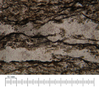Figure 1

Unfortunately, the cores from the G-1 borehole were lost to the beginning of the described study. Therefore, the detailed analysis of the rocks corresponding to the depth of the geophone was based on the samples from the region of Mt. Tolstyi Mys. According to [Sheimovich, 2000] these samples represent the rocks penetrated by the G-1 borehole at a depth of about 1000 m. Generally, the comparison shows that the data for the thin polished sections of these rocks (the analysis is carried out by M. Yu. Puzankov) agree with the results for the G-1 cores from the depths 734 to 1400 m. Inter alia, this conclusion is also valid for the pattern of the pore-fracture space. The results for the G-1 cores indicate that the density of the fractures and microcracks in the near-borehole area is quite high. Based on the analysis of the thin sections, the similar conclusion can also be drawn for the samples from Mt. Tolstyi Mys. The photo images of the thin polished sections of these samples (Figure 1) show quite large cracks filled with the milk-white quartz, which coexist with the abundant microcracks healed with brownish material. By analogy with the studies of the other rocks, it is assumed that this is a fine dispersed mixture of clay minerals, hydro-micas, and iron hydroxides. The diameter of the microcracks in the images is largely about $10^{-5}$ m. In the photos of the thin sections it can be seen that the directions and lengths of microcracks widely vary. Most often, the microcracks are rather long and they can be connected with each other.

Thus, the rocks of the G-1 borehole at a depth of about 1000 m, on one hand, are characterized by rather high density of the cracks, and, on the other hand, they have very low permeability for gaseous and liquid components. This situation is probably associated with the fact that most of the fractures and microcracks in the rock are healed, which results in the low fluid saturation of the pore-fracture space in this zone. At the same time, it should be noted that these results were obtained for the intact samples, which have not been damaged by the drilling operations. It can be assumed that the layer of the rocks immediately adjacent to the borehole, which is damaged by drilling, has a significantly higher permeability and higher density of the defects. The interpretation of the data on the permeability of the rocks in the zone of the G-1 borehole should take into account the fact that the coefficient of filtration for the liquid phase and the electrical conductivity of the rocks are linked with the geometrical parameters of the pore-fracture space by the different relationships. For example, as shown in [Titov, 2003], the dependence of the hydraulic permeability on the opening of a microcrack or on the diameter of a capillary is significantly stronger than the similar dependence of the electrical conductivity. Due to this, although not being the conductors for water, the narrow pores and capillaries can still remain the conductors for the electric current and play significant role in the processes associated with geoacoustic emission.

### Estimating the Probable Effects Associated With Piezoelectric Properties of the Rocks

The data on the cores described above indicate that the rocks in the G-1 borehole at the depths of the geoacoustic measurements contain significant amounts of quartz reaching 30% of the entire rock volume across the section. Due to this, it is important to estimate the probable implications of piezoelectric properties of the rocks for the results of geoacoustic measurements.

The sensitivity of the intensity of geoacoustic (acoustic) emission to the piezoelectric properties of the rocks under the action of external EM field was studied in a number of the laboratory experiments on the rock samples. The first experiments used artificial synthetic model specimens containing "piezoelectric" (quartz sand) and "non-piezoelectric" (diabase sand) additives to cement [Sobolev and Ponomarev, 2003]. The specimens were affected by bipolar rectangular EM pulses with frequencies of 250 Hz and 2.5 kHz and amplitude of 20 V.

The series of the experiments have shown that the rate of acoustic emission tends to increase (by about 1%) when the loaded specimen is affected by the EM impacts. No significant difference between the results for the "piezoelectric" and "non-piezoelectric" samples was observed. In the discussions of these results, the authors hypothesized that the low coefficient of mechanoelectrical transformation of the electrical energy supplied by the external EM impacts in the rocks ($10^{-5} - 10^{-6}$ [Sobolev and Demin, 1980]) is probably insufficient for causing the rate of the geoacoustic emission to increase via direct mechanoelectrical transformations.

Significant number of the experiments on the rock specimens, which make it possible to estimate the effects of piezoelectric properties of the rocks to their sensitivity to the external EM action, were carried out in 2002–2008 by the Research Station of the Russian Academy of Sciences in Bishkek, Kyrgyzstan (RS RAS). These experiments have shown that the presence of piezoelectric fraction in the rock affects the threshold intensity of the EM field that is sufficient to cause acoustoemission response of a specimen [Alad'ev et al., 2003; Bogomolov et al., 2004; Gavrilov and Bogomolov, 2008; Zakupin et al., 2003].

In the samples of piezoelectric materials, the acoustic responses to the external EM impacts occurred at significantly lower loads than in the samples without piezoelectric fraction, which indicates that the intensity of geoacoustic processes is controlled by the inverse piezoeffect. At the same time, the laboratory experiments show that distinct acoustic responses to the external EM impacts are also observed in the specimens that do not contain piezoelectric fraction when the external (relative) load is of about 0.75–0.95. This testifies to the existence of the mechanism of generation of the elastic field that is different from inverse piezoeffect. The experiments with the synthetic model specimens of piezoelectric rocks revealed the growth in the AE sensitivity of the samples with the increase in their water saturation [Zakupin, 2006]. This result, inter alia, supports the idea of generation mechanism of the elastic field that is different from inverse piezoeffect.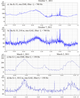Figure 2

The existence of such mechanism is also suggested by the presence of GAE responses to the external EM impacts identified in the measurements in the boreholes where the ambient rocks are practically void of piezoelectrical minerals. For example, these responses are detected in the R-2 borehole [Gavrilov et al., 2011] and the K-33 borehole located at a distance of 36 km from the G-1 borehole (Figure 2).

According to the drilling data, the geological section in the vicinity of the K-33 borehole in the depth interval from 80 to 300 m is represented by the alternation of andesite-basalt tuffs, tuff siltstones, tuff sandstones, basalts, andesite-basalts and diorite porphyrites and does not contain significant amounts of piezoelectric minerals [Report..., 1980]. The geoacoustic measurements in the borehole are conducted by the G-61 hydrophone installed at a depth of 210 m. The EM measurements are carried out with the use of underground electrical antenna whose main element is the coaxial cable with a length of 300 m, which is sunk into the borehole and which partially extends beyond the limits of the steel cased column. Generally, the EMI spectrum in the zone of the K-33 borehole is rather complex. Due to this, the synchronous geoacoustic and EM measurements in the K-33 are conducted within a narrow segment of the spectrum around the central frequency of 700 Hz, where the spectrum is mainly contributed by the natural EM sources.

The results of these measurements illustrated in Figure 2 show that the GAE responses to the external EMIs recorded in the K-33 and G-1 boreholes have commensurate relative amplitudes (normalized to the amplitudes of the EMI). The data shown in Figure 2 were obtained by the narrow-band digital filtering of the time series of the wideband EM and geoacoustic measurements. The central frequencies of the filters were 700 Hz for the data from the K-33 borehole and 1150 Hz for the data from the G-1. The geoacoustic measurements were carried out with the G-61 geophone in the K-33 borehole and with the A1612 geophone (vertical channel) in the G-1 borehole.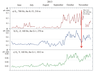Figure 3

The measurements also revealed commensurate GAE responses recorded in these two boreholes during the preparation and occurrence of the strong seismic events. The example in Figure 3 shows the variations in the envelopes of the relative rms intensities of GAE in the K-33 and G-1 boreholes in the time vicinity of the strong earthquake in Kamchatka. The data shown in Figure 3 were obtained by calculating the ratio $S_{\rm rl}= S_{\rm GAE}/S_{\rm EMI}$, where $S_{\rm GAE}$ and $S_{\rm EMI}$ are the areas below the envelopes of rms intensities of GAE and EMI signals, respectively, on the same time interval with a length of a few hours during each day.

The analysis of the long time series of the data provided by the combined measurements in the boreholes within the Petropavlovsk-Kamchatsky geodynamical testing area also suggests that the composition of the rocks in the borehole environment is not a primary factor governing the geoacoustic sensitivity of the rocks to the EMI. For instance, a significantly larger contribution is provided by the water saturation of the rocks, which depends on the stress-strain state of the geological medium. According to the data presented in [Gavrilov, 2013; Gavrilov et al., 2014], the amplitudes of GAE responses recorded in the G-1 borehole 3.5 months after the Tohoku mega-earthquake (March 11, 2011, $M = 9.0$) have decreased by about a factor of 20 due to the decrease in the fluid saturation of the rocks. Thus, both the in situ measurements and the laboratory experiments suggest that modulation of the intensity of GAE by the external EMI can be rendered by the physical mechanisms that are different from the inverse piezoeffect, which is typical of the most rocks.

### The Structure of the Double Electrical Layer at the Boundary Between the Solid and Liquid Phases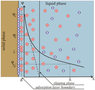Figure 4

The processes of interaction between the solid and liquid phases necessarily include the electrical double layers, which are located at the interfacial boundary and composed of the spatially separated electric charges. The generation of EDL in the fluid-saturated rocks is primarily associated with the existing imbalance between the chemical potentials of the components (ions) in the solid and liquid phases. This imbalance produces a difference in the electric potential on the interfacial boundaries, which is accompanied by the separation of the electrical charges. The modern understanding of the structure of EDL is based on the Gouy-Chapman-Stern model [Salem, 2003], which is schematically illustrated in Figure 4. EDL is composed of a layer of the potential-determining ions (PDI), which are rather firmly anchored to the surface of the solid phase, and the layer of the ions of the same absolute charge but opposite sign (counterions) located in the liquid phase. Thus, the PDI layer forms the inner part of EDL whereas the layer of counterions forms its outer part. In the rocks, the solid phase is typically charged negatively, and the liquid phase carries a positive charge. It can be assumed that PDI are located in the same plane with the surface of the solid phase. In contrast to the PDI, which are localized within the surface, the counterions are distributed throughout the volume of the liquid phase. One part of the counterions is directly adjacent to the interfacial surface due to chemical adsorption and electrostatic forces and it forms a dense adsorption layer. The ions of the adsorption layer are firmly associated with the interphase boundary. The thickness of the adsorption layer $\delta$ is nearly equal to the diameter of the hydrated ions that compose this layer ($\approx 0.3 \div 0.4$ nm).

The other part of the counterions is located in the diffuse layer, which contains ion species of the both signs (counterions are prevailing) and is only kept near the surface by the electrostatic (Coulomb) forces. The plane dividing the adsorption layer from the diffuse layer is referred to as the Stern plane. The diffuse layer consists of the fixed and mobile parts separated by the slipping plane, beyond which ion motion in the diffuse layer becomes possible. According to the present-day understanding, the fixed part of EDL generally comprises a layer of immobile water with a thickness of one to a few molecular layers, which is directly adjacent to the interfacial surface. During the relative motion of the phases, this layer moves together with the solid phase. In the adsorption layer (just as in a flat capacitor), the electric potential linearly decreases. In the diffusion layer, the potential diminishes exponentially due to the decrease in the concentration of counterions with the increasing distance to the interfacial boundary caused by their thermal motion:

\begin{eqnarray*} \varphi_x = \varphi_{\delta} e^{- \chi x} \end{eqnarray*}

where $x$ is the distance from the Stern plane to a point in the liquid phase; $\varphi_{\delta}$ is the potential of the Stern plane; and $\chi$ is Debye parameter. Quantity $1/\chi = \lambda$ is the effective thickness of the diffuse layer, i.e. the distance at which the potential decreases by a factor of e relative to the value $\varphi_{\delta}$. The effective thickness of the diffuse layer can be sufficiently accurately calculated by the formula [Damaskin and Petrii, 1987]:

\begin{equation} \tag*{(1)}\label{1} \lambda = \frac{1}{zF} \sqrt{\frac{\varepsilon_0 \varepsilon RT}{2 C_0}} \end{equation}

where $z$ is the valence of ions; $F=9.65 \times 10^4$ [C/mol] is the Faraday constant; $\varepsilon_0 =8.85 \times 10^{-12}$ is the electrical constant (electric permittivity of free space) [F/m]; $\varepsilon =81$ is the dielectric permittivity of free water; $k_b = 1.38 \times 10^{-23}$ is the Boltzmann's constant [J/K]; $R=8.31$ [J/mol K] is the universal gas constant; $T$ is absolute temperature of the fluid [deg]; $C_0$ is the equilibrium ion concentration in a free solution [mol/m$^3$]; $e = 1.602 \times 10^{-19}$ is the electron charge [C].

According to the results of hydrogeochemical studies, water in the G-1 borehole has mainly sodium chloride ion composition [Khatkevich and Ryabinin, 2006], due to which the fluid in the calculations can be treated as a binary electrolyte. The average water mineralization in the G-1 borehole is 12.3 g/l. The temperature estimates at the depth of the geophone in this borehole give $T=20$° C. With these values and thickness of the adsorption layer of about 0.3 nm, (1) provides the estimate of the total thickness of EDL at about 1 nm.

### The Probable Physical Mechanism of Modulation of the Intensity of Geoacoustic Processes by the External EMI

Let us qualitatively consider the probable physical mechanism responsible for the modulation of the intensity of GAE by the varying external EM field. We disregard the motion of the pore fluid associated with the redistribution of the pore pressure in the geological medium, and take into account the following considerations. Firstly, according to the present-day understanding, GAE is primarily associated with the noise produced by the processes of repacking that occur in the grained geological medium under the action of mechanical stresses. Secondly, the geological section in the zone of the G-1 borehole at the depth of the main geoacoustic measurements (about 1000 m) is largely composed of highly resistive dense rocks, whose electric resistivity at low moisture (up to 4%) is strongly sensitive to the variations in the fluid saturation.

As shown in [Parkhomenko, 1965; Parkhomenko and Bondarenko, 1960], the variations in the fluid saturation (moisture) of the rocks up to a few fractions of the percent in most cases cause the electric resistivity to change by the order of magnitude and even stronger. For example, the drop in the moisture of basalt from 0.49% to 0.26% increases the resistivity from $9.0\times 10^7$ to $3.1 \times 10^9$ Ohm cm. For siltstone, the decrease in the moisture from 0.54% to 0.44% results in the increase in resistivity from $1.5 \times 10^6$ to $8.4 \times 10^8$ Ohm cm. The EM measurements in the G-1 borehole with the use of the underground electrical antenna described in [Gavrilov, 2013] support these estimates.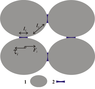Figure 5

Taking into account the character of the rocks in the zone of the G-1 borehole and the presumed origin of GAE, we use the model of a non-water-saturated grained medium (Figure 5), where a film water surrounding the grains forms the narrow electrically conductive channels with a length $l_2$, and the wide channels with a length $l_1$ are formed by water at the contacts between the grains [Titov, 2003; Titov and Tarasov, 2001].

Let the $i$-th grain be affected by the mechanical force $F_i$, which is parallel (tangential) to the interfacial boundary. Since a liquid in narrow capillaries behaves as a viscoplastic body, which has a shear resistance [Mironenko, 2005], the motion of the grain under the action of force $F_i$ is possible if

\begin{eqnarray*} \tau_i - F_i <0 \end{eqnarray*}

where $\tau_i$ is the force of viscous friction for EDL of the $i$-th grain.

If there is an electric field in the medium, then each arbitrary element of the pore liquid with a volume $dV$ is affected by force $\boldsymbol{\rm E} \rho dV$, where $\boldsymbol{\rm E}$ is the strength of the acting electric field, $\rho$ is the volume charge density for the considered element of the volume. In the steady-state (stationary) conditions, the volumetric force $\boldsymbol{\rm E} \rho dV$ is balanced by the forces of viscous friction acting on the surface that limits this element of the volume.

For simplicity, we consider the one-dimensional (1D) problem in which the capillary is modeled by a flat wide (compared to the thickness of EDL) pore with the infinitely small thickness $dx$ and with the unit areas of the large sides (faces). For this case, $dV=dx$, and the balance condition between force $\boldsymbol{\rm E} \rho dV$ and viscous friction is reduced to the equality

\begin{equation} \tag*{(2)}\label{2} d\tau = -E \rho dx = - Edq \end{equation}

where $d \tau$ is the difference between the friction forces on the farther (according to $x$) and nearer sides of the plane layer; $q$ is the charge of the considered element of the volume [Simanova, 2004]. Integration of (2) gives

\begin{eqnarray*} \tau (x) = -E q (x) \end{eqnarray*}

where $\tau (x)$ is the force of viscous friction for a certain plane that is parallel to the surface of the solid phase and spaced by a distance $x$ apart from it; $q(x)$ is the charge of the part of the diffuse layer of EDL that is located outside this plane.

Let the varying external electric field $E_{\rm ex}$ acts on the studied element of the volume of the geological medium along the normal direction to the interfacial surface and be sinusoidal with zero initial phase: $E(t)_{\rm ex}= E_m \sin \omega t$, where $\omega =2 \pi f$ is the angular frequency of the signal. Taking into account the electrostatic (Coulomb) field of EDL, we obtain the following formula for the resulting electric field acting on the element of a liquid:

\begin{eqnarray*} E = E_0 + E_m \sin \omega t \end{eqnarray*}

where $E_0 = -\chi \varphi_0 \exp(- \chi x)$ is the electrostatic field of EDL for the studied element of the volume of the liquid, $\varphi_0$ is the surface potential, and $x$ is the distance along the normal from the surface of the solid phase.

Under the action of the varying electric field, the charge of the external part of EDL will vary with time. We estimate the changes in the charge $q(t)$ using the model of EDL in the form of a single flat micro-capacitor [Antropov, 1984; Damaskin and Petrii, 1987]. One plate of this capacitor is formed by the layer of PDI on the surface of the solid phase, and the other plate is composed of the layer of counterions, which is located in the liquid. The pore-fracture space is represented by a set of the tubes of current with a cross-section $S$, each of which contains EDL on the interfacial surface. For this model, the value of the varying component of the current flowing through EDL is identical at each point of the circuit. The displacement current $I_{\rm dis}$ flowing through the capacitor is in this case equal in the absolute value to the conduction current $I_{\rm dis} = I_E$ and

\begin{eqnarray*} \frac{d q}{d t} = S \sigma E_{\rm ex} \end{eqnarray*}

where $\sigma$ is the electric conductivity of a liquid fluid in the pore-fracture space and $q$ is the charge on the plates of the microcapacitor. After the integration, we obtain:

\begin{equation} \tag*{(3)}\label{3} q(t) = q_0 (x)- \frac{\sigma S E_m \sin (\omega t - \pi/2)}{\omega} \end{equation}

where $q_0(x)$ is the charge of the external part of EDL, which is not associated with the external electric field.

Formula (3) shows that under the influence of the external harmonic EM impact, the charge of the external part of EDL will vary in time within $\pm (S \sigma E_m)/\omega$ with the frequency of the EM impact $\omega$ and with a phase delay of $\pi/2$. In this case, the time variations of the force of friction $\tau(t)$ between the mobile part of the liquid fluid and the surface of the solid phase of EDL will also be related to the parameters of the external electric field and electric conductivity of the fluid. The charge $q_0(x)$ can be obtained by integrating the quantity $\rho dx$. Since we analyze the problem in one-dimensional model, we can use the 1D Poisson equation

\begin{eqnarray*} \frac{d^2 \varphi}{d x^2} = - \frac{\rho}{\varepsilon \varepsilon_0} \end{eqnarray*}

and then we obtain

\begin{eqnarray*} \rho = - \varepsilon \varepsilon_0 \frac{d^2 \varphi}{d x^2}, \qquad q_0 (x) = \varepsilon \varepsilon_0 \frac{d \varphi}{d x} \end{eqnarray*}

For the slipping plane located at distance $s$ from the surface of the solid phase, the variations in the friction force $\tau_s$ under the action of the external harmonic EM impact are expressed by the following formulas

\begin{eqnarray*} \tau_s(t) = (E_{s0} + E_m \sin \omega t) (q_{s0} - \frac{\sigma S E_m \cos \omega t}{\omega} )\approx \end{eqnarray*} \begin{eqnarray*} q_{s0} E_{s0} + q_{s0} E_m \sin \omega t + \frac{\sigma S E_{s0} E_m \sin (\omega t + \pi/2)}{\omega} = \end{eqnarray*}

\begin{equation} \tag*{(4)}\label{4} q_{s0} E_{s0} + A \sin (\omega t -\psi) \end{equation}

where $E_{s0}$ is the strength of the Coulomb field of EDL at a distance s from the surface of the solid phase; $q_{s0}$ is the charge outside the selected plane, which is not associated with the varying external electric field. Quantities $A$ and $\psi$ are determined by the following expressions:

\begin{eqnarray*} A= \sqrt{q^2_{s0} + \Bigl( \frac{\sigma S E_{s0} E_m}{\omega} \Bigr)^2} \end{eqnarray*} \begin{eqnarray*} \sin \psi = \frac{\sigma S E_{s0} E_m} {\omega \sqrt{(q_{s0} E_m)^2 + (\sigma S E_{s0} E_m/\omega)^2}} \end{eqnarray*} \begin{eqnarray*} \cos \psi = \frac{q_{s0} E_m} { \sqrt{(q_{s0} E_m)^2 + (\sigma S E_{s0} E_m/\omega)^2}} \end{eqnarray*}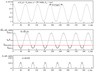Figure 6

From (4) is can be seen that under the action of the external harmonic EM impact, the friction between the mobile part of the liquid phase and the surface of the solid material will oscillate (vary) about the average value $q_{s0}E_{s0}$ with the frequency of the EM impact and with the phase lag $\psi$. The amplitude of the variations in the friction force will depend on the electric conductivity of the liquid fluid, the intensity of the electric field, and the frequency of the external EMI. Each single act of motion of a grain can be treated as a manifestation of the acoustic emission caused by the local dynamical rearrangement of the structure of the material (Figure 6). On the scale of macroscopic volume of the geological medium, the periodical decrease in the force of friction $\tau_i$ is favorable for the increase in the number of the acts of motion of the solid phase relative to the fluid phase under the action of the mechanical stresses associated with tectonic strains, lithostatic pressure, and influences of seismic waves and tides. It is important that for the real geological medium, the variations in $\tau_i$ caused by the action of a weak harmonic electric field with the intensity of a few mV/m for each single EDL is extremely low compared to the action of the electrostatic field of EDL (by ten orders of magnitude and even lower). Due to this, these effects can only be studied with highly sensitive geoacoustic measurements, which can typically be achieved by placing the instruments into the boreholes at a depth of at least 200 m. The high sensitivity of such measurements is primarily associated with the significant reduction of the industrial noise whose sources are located on the surface. For example, according to the geoacoustic measurements in the G-1 borehole at a depth of about 1000 m, the surface noise at these depths is attenuated by more than two orders of magnitude [Gavrilov et al., 2006].

The geoacoustic signal recorded by the borehole measurements is, in fact, composed by the superimposition of the radiation from a very large number of the point sources of GAE, which are simultaneously acting at the different points of the "noise" zone. The estimates of the size of the noise zone that determines the bulk (about 90%) of the recorded GAE show that in the case of measurements in a sufficiently deep borehole, this zone for the frequencies of about 150–160 Hz is a sphere with a radius of at least a few hundred of meters. In [Lutikov, 1992], the radius $R_{\rm ef}$ of the noise zone is approximately estimated by the formula $R_{\rm ef} \approx Q \times \lambda$, where $Q$ is the $Q$-factor of the medium and $\lambda$ is the wavelength. In this case, for GAE at a frequency of about 160 Hz and with the $Q$-factor in the borehole zone $Q\approx 100$, $R_{\rm ef} \approx 1200 - 1300$ m. This interval of $R_{\rm ef}$ almost coincides with the estimates presented in [Khavroshkin, 1999]. At the same time, it is worth noting that in the real conditions, the radius of the zone that provides the main contribution to the value of recorded GAE can be significantly smaller than the calculated estimate due to the specificity of the rocks in the near-borehole zone, where the geological medium is marked with highly dense defects and increased permeability due to drilling.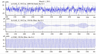Figure 7

Nevertheless, the noise zone is in any case quite large and, therefore, the recorded GAE signal is composed of fairly many simultaneous geoacoustic pulses from separate point sources due to restructuring of the field of mechanical stresses. From the standpoint of the theory of random processes, the recorded geoacoustic signal in this case is a result of summation of instantaneous values over a very large ensemble of the sample functions. Due to this, the signal-to-noise ratio increases and achieves the level that is sufficient for identifying the effects of modulation of GAE by the continuous weak EMI. In the case of wideband recording, the GAE signal is generally a sequence of the overlapping or closely juxtaposed separate pulses. This is clearly seen in Figure 7a, which presents the typical example of a short (about 1 s) realization of GAE data.

### The Variations in F$_i$(t) Due to the Action of the Harmonic Field With Slowly Varying Intensity Amplitude

The 1-s realization shown in Figure 7 is selected from the 1.5-h time series illustrated in Figure 2. The graph in Figure 7c shows that the intensity of the external EMI remains almost constant on this short time interval, and the data in Figure 7 can be considered as the example of the geoacoustic response of a macroscopic domain in the geological medium to the action of the harmonic electric field having constant intensity amplitude. The comparison of the data shown in Figure 2 and Figure 7 illustrates the fact that in order for the effects of modulation of GAE by the external EMI be reliably identified, the duration of the measurements should correspond to the characteristic period of variations in the intensity of EMI.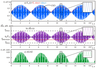Figure 8

The main results of the simultaneous geoacoustic and EM measurements at the Petropavlovsk-Kamchatsky geodynamical testing area were obtained during the study of the response of the geological medium to the EM impacts having a diurnal periodicity. Therefore, consider the situation when the intensity of the external electric harmonic field $E_{\rm ex}(t) = E_m \sin \omega t$ slowly varies with time: $E_m= E_s \sin \Omega t$, $\Omega \ll \omega$. Figure 8a illustrates the results of calculating the friction force $\tau_i$ in the EDL that is affected by the external harmonic electric field whose intensity $E_m$ sinusoidally varies from 0.2 mV/m to 1.0 mV/m with a period of 24 h: $E_{\rm ex}(t) = E_m \sin \omega t$, $E_{m} = E_s \sin \Omega t$, $\omega =2\pi \times 160$ Hz, $\Omega=7.27 \times 10^{-5}$ Hz. This pattern of electromagnetic influence is characteristic of the G-1 borehole. The presented data show that with this character of EMI, the force of friction is modulated in accordance with the law of variation of the external EM forcing: $E_{m} = E_s \sin \Omega t$. For each sufficiently short time interval on which the value of $E_m$ can be assumed to be constant, the variation in the friction force for EDL will correspond to the case of the harmonic electric impact with constant amplitude considered above.

On the scale of the macroscopic volumes of the geological medium, the characteristic periodicity for these short intervals is the variation in the friction force $\tau_i$ with frequency $\omega$ (see the inset in Figure 8b). On the intervals with a length $T \geq 2 \pi /\Omega$, the amplitude of the external electric field $E(t)_{\rm ex}$ is sensitive to the current values of $E_{m} = E_s \sin \Omega t$, which manifests itself by the modulation of the amplitude of force $\tau_i$ with frequency $\Omega$ (Figure 8b). In accordance with the variations in the friction force $\tau_i$, the increments in the intensity of GAE on the scale of the macroscopic volume of the geological medium on these time intervals will also be modulated with frequency $\Omega$ (Figure 8c).

### Conclusions

1. The borehole measurements analyzed in this study support the previous conclusions suggesting that the EM modulation of GAE can be rendered by the physical mechanisms that are different from the inverse piezoelectric effect.

2. The key role in the mechanism of modulation of the intensity of geoacoustic processes by the external audio-frequency EM field is played by the EDL at the interfacial boundaries between the solid and liquid phases in the fluid-saturated medium.

3. The theoretical estimates based on a simple EDL model show that the modulating effect of the weak audio-frequency EM fields on the intensity of GAE recorded in the boreholes is due to the variations in the forces of viscous friction between the mobile part of the liquid fluid and the surface of the solid phase of EDL. The variations in the forces of viscous friction occur with the frequency of the external EM field and with the amplitude that is proportional to the amplitude of the external EM field. On the scale of a macroscopic volume of the geological medium, the periodical decrease in the friction forces facilitates the increase in the number of the acts of motion of the solid phase relative to the liquid fluid under the influence of the acting mechanical stresses.

4. The comparison of the obtained theoretical estimates with the results of multi-year measurements at the Petropavlovsk-Kamchatsky geodynamical testing area demonstrates their close qualitative agreement.

In conclusion, it is worth noting that due to the complex structure of the rocks and processes occurring in the geological medium, the relationships between the intensity of the geoacoustic processes and the variations in the intensity of the external EMI only allow of a largely qualitative description. The adequacy of the suggested theoretical models for the real situations can, to a certain extent, be estimated by the criterion of their correspondence to the results of the borehole measurements in the in situ rocks in the natural geological environment. In this respect, it is remarkable that the theoretical models suggested in the present study qualitatively agree with the results of the borehole measurements at the Petropavlovsk-Kamchatsky geodynamical testing area.

#### Acknowledgments

I am grateful to A. V. Naumov and I. A. Panteleev for their valuable discussions and constructive helpful advices. The work was supported by the Russian Foundation for Basic Research (grant 12-05-00670-a), the Presidium of the Far East Branch of the Russian Academy of Sciences (grant 12-III-A-08-167), and the Program for the State Support of the Leading Scientific Schools of the Russian Federation (grant NSh-5583.2012.5).

### References

Alad'ev, A. V., et al. (2003), The regularities of the acoustic emission in the samples of geomaterials tested for creep under energy loading by physical fields, Problems of Seismology in the Third Millennium, SO RAN, Novosibirsk, 168–172.

Antropov, L. I. (1984), Theoretical Electrochemistry, Vysshaya shkola, Moscow.

Bogomolov, L. M., et al. (2004), Acoustic emission response of rocks to electric power action as seismic-electric effect manifestation, Ann. Geophysics, 47, 1, 65–72.

Damaskin, B. B., and O. A. Petrii (1987), Electrochemistry, Vysshaya shkola, Moscow.

Gavrilov, V. A. (2007), Physical causes of diurnal variations in the geoacoustic emission level, Dokl. Earth Sci., 414, 4, 638–641, doi:10.1134/S1028334X07040320.

Gavrilov, V. A. (2013), On the method for continuous monitoring the electric resistivity of the rocks, Seismich. Instr., 49, 3, 25–38.

Gavrilov, V. A., and L. M. Bogomolov (2008), On the question of the influence of natural electromagnetic fields on the rate of geoacoustic emission, Geophysical Monitoring and Problems of Seismic Safety of the Russian Far East: Proc. Regional Scientific and Technical Conf., Petropavlovsk-Kamchatsky, November 11–17, 2007, Petropavlovsk-Kamchatsky, 59–63.

Gavrilov, V., L. Bogomolov, Yu. Morozova, and A. Storcheus (2008), Variations in geoacoustic emissions in a deep borehole and its correlation with seismicity, Ann. Geophysics, 51, 5/6, 737–753.

Gavrilov, V. A., L. M. Bogomolov, and A. S. Zakupin (2011), Comparison of the geoacoustic measurements in boreholes with the data of laboratory and in-situ experiments on electromagnetic excitation of rocks, Izv. Phys. Solid Earth., 47, 11, 1009–1019, doi:10.1134/S1069351311100041.

Gavrilov, V. A., Yu. V. Morozova, and A. V. Storcheus (2006), Variations of geoacoustic emission rate in the deep G-1 borehole (Kamchatka) and their correlation to seismic activity, Vulkanol. Seismol., 1, 52–67.

Gavrilov, V. A., I. A. Panteleev, G. V. Ryabinin, and Yu. V. Morozova (2013), Modulating impact of electromagnetic radiation on geoacoustic emission of rocks, Russ. J. Earth Sci., 13, ES1002, doi:10.2205/2013ES000527.

Gavrilov, V. A., I. A. Panteleev, and G. V. Ryabinin (2014), The physical basis of the effects caused by electromagnetic forcing in the intensity of geoacoustic processes, Izv. Phys. Solid Earth, 50, 1, 87–101, doi:10.1134/S1069351314010042.

Khavroshkin, O. B. (1999), Some Problems of Nonlinear Seismology, OIFZ RAN, Moscow.

Khatkevich, Yu. M., and G. V. Ryabinin (2006), Hydrogeochemical studies in Kamchatka associated with searching for the precursors of the earthquakes, Vulkanol. Seismol., 4, 34–42.

Lutikov, A. I. (1992), Estimate of the effective radius of the zone controlled by the sources of endogenous microseismic noise, Vulkanol. Seismol., 4, 111–115.

Mironenko, V. A. (2005), Dynamics of the Ground Water, MGGU, Moscow.

Parkhomenko, E. I. (1965), Electric Properites of Rocks, Nauka, Moscow.

Parkhomenko, E. I., and A. T. Bondarenko (1960), Influence of unidirectional pressure on electric resistivity of rocks, Izv. Akad. Nauk SSSR, Ser. Geofiz., 2, 326–332.

Report (1980), On the prospecting and exploration at the Verkhnyaya Paratunka hydrothermal field, Termal'nyi village, Kamchatka: Paratunskaya hydrogeological expedition of Kamchatka PGO, vol. 4, Mingeo SSSR.

Report (1988), On the thermal water drilling at the wildcat G-1 well on the Khlebozavod segment of the Petropavlovskaya area in 1986–1988, Petropavlovsk-Kamchatsky.

Salem, S. S. (2003), Theory of Electrical Double Layer, Moscow: Fizmatlit.

Simanova, S. A. (Ed.) (2004), New Handbook of the Chemist and Technologist. Electrode Processes. Chemical Kinetics and Diffusion. Colloidal Chemistry, Professional, St. Petersburg.

Sheimovich, V. S., and A. B. Markovskii (Ed.) (2000), The 1:200 000 State Map of the Russian Federation, South Kamchatka Series. Sheets N-57-XXI (Northern Koryaki), N-57XXVII (Petropavlovsk-Kamchatsky), N-57-XXXIII (Mt. Mutnovsakaya), Explanatory Note, Moscow.

Sobolev, G. A., and V. M. Demin (1980), Mechanoelectrical Events on the Earth, Nauka, Moscow.

Sobolev, G. A., and A. V. Ponomarev (2003), Earthquake Physics and Precursors, Nauka, Moscow.

Titov, K. V. (2003), Electrokinetic phenomena in rocks and its application to geoelectrics, Doct. (Geol.-Min.) Dissertation, A. A. Logachev All-Russian Research Institute of Exploration Geophysics (VIRG Rudgeofizika), St. Petersburg.

Titov, K. V., and V. A. Tarasov (2001), Time constants of induced polarization in water-saturated sands: theory and experiments, Russian Geology and Geophysics, 42, 6, 988–995.

Vlasov, Yu. A., V. A. Gavrilov, V. P. Denisenko, and O. V. Fedoristov (2007), Telemetric system of the multiinstrumental network for geophysical monitoring, Seismicheskie Pribory, 44, 2, 32–38.

Vsevolozhskii, V. A. (2007), Basics of Hydrogeology, Moscow, MSU.

Zabarnyi, G. N., A. B. Burganov, and G. M. Gaidarov (1990), The results of the prospecting for geothermal resources in Petropavlovsk-Kamchatsky, Kamchatka Complex Department of VNIPIGeoterm, Petropavlovsk-Kamchatsky.

Zakupin, A. S., et al. (2003), The influence of the external electromagnetic field on the activity of acoustic emission in loaded geomaterials, Geodynamics and Geoecological Problems of the High-Mountain Regions, Yu. G. Leonov and S. V. Gol'din (Eds.), Moscow–Bishkek, 304–324.

Zakupin, A. S. (2006), The study of the electrically triggered variations in the acoustic emission under the deformation of the samples of the geological materials, Extended Abstract of the Cand. (Phys.-Math.) Dissertation, Schmidt Institute of Physics of the Earth of the Russian Academy of Sciences, Moscow.

Received 25 September 2014; accepted 16 October 2014; published 31 October 2014.Citation: Gavrilov V. A. (2014), On the mechanism of intensity variations of geoacoustic emission caused under the action of audio-frequency electromagnetic field, Russ. J. Earth Sci., 14, ES2003, doi:10.2205/2014ES000541.

Generated from LaTeX source by SemTeXML, v.1.2 software package.